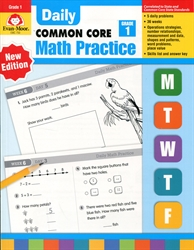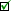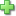# Daily Math Practice Grade 1

Current Retail Price: \$22.99
Used Price: \$12.00 (1 in stock)Condition Policy

See series description for full review.

The skills practiced in Daily Math Practice, Grade 1 include:

Computation

• subtraction facts
• word problems

Number

• write number sentences
• write word problems
• read & write number words
• place value
• count by two, fives, tens
• odd/even
• ordinal numbers
• estimation
• greater/less than, equal to
• properties, number relationships
• fractions

Patterns

• sort & classify
• create, name, describe, extend

Geometry

• shapes
• symmetry

Measurement

• weight and capacity
• time
• money

DataClick here to write a review